Test: Kirchhoff’s Current & Voltage Law

# Test: Kirchhoff’s Current & Voltage Law

Test Description

## 20 Questions MCQ Test Basic Electrical Technology | Test: Kirchhoff’s Current & Voltage Law

Test: Kirchhoff’s Current & Voltage Law for Electrical Engineering (EE) 2023 is part of Basic Electrical Technology preparation. The Test: Kirchhoff’s Current & Voltage Law questions and answers have been prepared according to the Electrical Engineering (EE) exam syllabus.The Test: Kirchhoff’s Current & Voltage Law MCQs are made for Electrical Engineering (EE) 2023 Exam. Find important definitions, questions, notes, meanings, examples, exercises, MCQs and online tests for Test: Kirchhoff’s Current & Voltage Law below.
Solutions of Test: Kirchhoff’s Current & Voltage Law questions in English are available as part of our Basic Electrical Technology for Electrical Engineering (EE) & Test: Kirchhoff’s Current & Voltage Law solutions in Hindi for Basic Electrical Technology course. Download more important topics, notes, lectures and mock test series for Electrical Engineering (EE) Exam by signing up for free. Attempt Test: Kirchhoff’s Current & Voltage Law | 20 questions in 20 minutes | Mock test for Electrical Engineering (EE) preparation | Free important questions MCQ to study Basic Electrical Technology for Electrical Engineering (EE) Exam | Download free PDF with solutions
 1 Crore+ students have signed up on EduRev. Have you?
Test: Kirchhoff’s Current & Voltage Law - Question 1

### Find the value of v if v1 = 20V and value of current source is 6A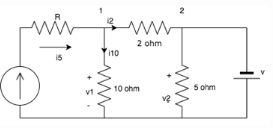Detailed Solution for Test: Kirchhoff’s Current & Voltage Law - Question 1

The current through the 10 ohm resistor = v1 / 10 = 2A

Applying KCL at node 1: i5 = i10 + i2

i= 6 - 2 = 4A

Thus the drop in the 2 ohm resistor = 4×2 = 8V

v1 = 20V; hence v2 = 20-v across 2 ohm resistor = 20 - 8 = 12V
v2 = v since they are connected in parallel.
v = 12V

Test: Kirchhoff’s Current & Voltage Law - Question 2

### Calculate the current A.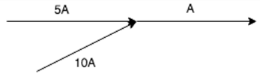Detailed Solution for Test: Kirchhoff’s Current & Voltage Law - Question 2

KCL states that the total current leaving the junction is equal to the current entering it. In this case, the current entering the junction is 5 A + 10 A = 15A

Test: Kirchhoff’s Current & Voltage Law - Question 3

### Calculate the current across the 20 ohm resistor.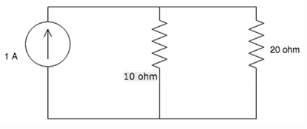Detailed Solution for Test: Kirchhoff’s Current & Voltage Law - Question 3

Assume lower terminal of 20 ohm at 0V and upper terminal at V volt and applying KCL, we get V/10 + V/20 = 1

V = (20 / 3) V

So, current through 20 ohm = V/20 = (20/3) / 20 = 1/3 = 0.33V

Test: Kirchhoff’s Current & Voltage Law - Question 4

Calculate the value of I3, if I= 2A and I= 3A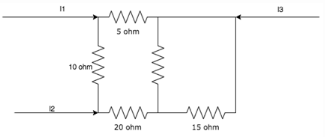Detailed Solution for Test: Kirchhoff’s Current & Voltage Law - Question 4

According to KCL, I+ I+ I= 0

Hence I= - (I+ I2) = - 5A

Test: Kirchhoff’s Current & Voltage Law - Question 5

Find the value of i2, i4 and i5 if i= 3A, i= 1A and i= 1A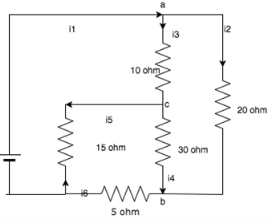Detailed Solution for Test: Kirchhoff’s Current & Voltage Law - Question 5
•  At junction a: i- i3 - i2 = 0
i= 2A
• At junction b: i+ i- i= 0
i= -1A
• At junction c: i- i+ i= 0
i= 2A
Test: Kirchhoff’s Current & Voltage Law - Question 6

What is the value of current if a 50C charge flows in a conductor over a period of 5 seconds?

Detailed Solution for Test: Kirchhoff’s Current & Voltage Law - Question 6

Current = Charge/Time

Here charge = 50C and time = 5 seconds

so current = 50/5 = 10A

Test: Kirchhoff’s Current & Voltage Law - Question 7

KCL deals with the conservation of?

Detailed Solution for Test: Kirchhoff’s Current & Voltage Law - Question 7

KCL states that the amount of charge entering a junction is equal to the amount of charge leaving it, hence it is the conservation of charge.

Test: Kirchhoff’s Current & Voltage Law - Question 8

KCL is applied at _________

Detailed Solution for Test: Kirchhoff’s Current & Voltage Law - Question 8

KCL states that the amount of charge leaving a node is equal to the amount of charge entering it, hence it is applied at nodes.

Test: Kirchhoff’s Current & Voltage Law - Question 9

KCL can be applied for __________

Detailed Solution for Test: Kirchhoff’s Current & Voltage Law - Question 9

KCL is applied for different nodes of a network whether it is planar or non-planar.

Test: Kirchhoff’s Current & Voltage Law - Question 10

What is the value of the current I?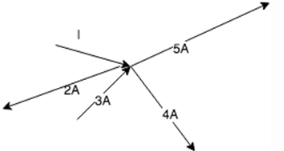Detailed Solution for Test: Kirchhoff’s Current & Voltage Law - Question 10

At the junction, I - 2 + 3 - 4 - 5 = 0

Hence I = 8A

Test: Kirchhoff’s Current & Voltage Law - Question 11

Calculate the value of V1 and V2.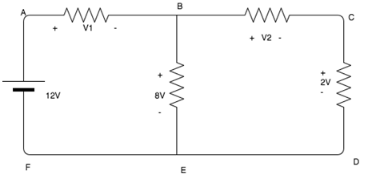Detailed Solution for Test: Kirchhoff’s Current & Voltage Law - Question 11

Using KVL, 12 - V1 - 8 = 0.

V1 = 4V

8 - V2 - 2 = 0

V2 = 6V

Test: Kirchhoff’s Current & Voltage Law - Question 12

KCL deals with the conservation of?

Detailed Solution for Test: Kirchhoff’s Current & Voltage Law - Question 12

KCL states that the amount of charge entering a junction is equal to the amount of charge leaving it, hence it is the conservation of charge.

Test: Kirchhoff’s Current & Voltage Law - Question 13

Calculate the voltage across the 10 ohm resistor.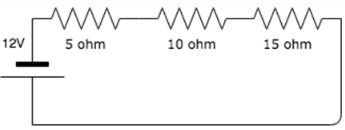Detailed Solution for Test: Kirchhoff’s Current & Voltage Law - Question 13

Using voltage divider rule, V = 10*12/30 = 4V

Test: Kirchhoff’s Current & Voltage Law - Question 14

Find the value of the currents I1 and I2.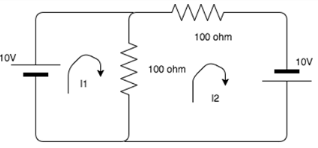Detailed Solution for Test: Kirchhoff’s Current & Voltage Law - Question 14

Using KVL, the matrix to find the loop currents are:
MATRIX 10 -100 * i1 + 100 * i2 = 0
MATRIX  10 - 200 * i2 + 100 * i1 = 0
I1 = 0.3, I2 = 0.2

Test: Kirchhoff’s Current & Voltage Law - Question 15

The sum of the voltages over any closed loop is equal to __________

Detailed Solution for Test: Kirchhoff’s Current & Voltage Law - Question 15

According to KVL, the sum of the voltage over any closed loop is equal to 0.

Test: Kirchhoff’s Current & Voltage Law - Question 16

What is the basic law that has to be followed in order to analyze any circuit?

Detailed Solution for Test: Kirchhoff’s Current & Voltage Law - Question 16

Kirchhoff’s laws, namely Kirchhoff’s Current Law and Kirchhoff’s Voltage law are the basic laws in order to analyze a circuit.

Test: Kirchhoff’s Current & Voltage Law - Question 17

Every____________ is a ____________ but every __________ is not a __________

Detailed Solution for Test: Kirchhoff’s Current & Voltage Law - Question 17

According to Kirchhoff’s Voltage Law, Every mesh is a loop but every loop is not a mesh.

Test: Kirchhoff’s Current & Voltage Law - Question 18

Solve for I.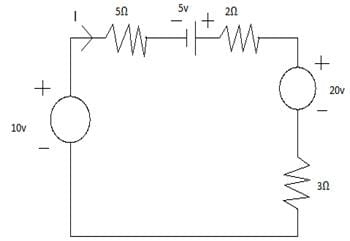Detailed Solution for Test: Kirchhoff’s Current & Voltage Law - Question 18

Veq = 10 + 5 -20 = -5u
Req = 5 + 2 + 3 = 10Ω
I = V/R = -5/10 = -0.5A.

Test: Kirchhoff’s Current & Voltage Law - Question 19

Calculate VAB.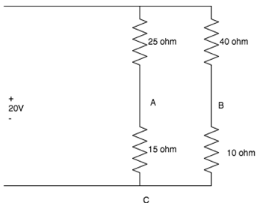Detailed Solution for Test: Kirchhoff’s Current & Voltage Law - Question 19

For branch A: VAC= 15 * 20 / (25+15) = 7.5V
For branch B: VBC= 10 * 20 / (10+40) = 4V
Applying KVL to loop ABC
VAB+VBC+VCA = 0
VAB = 3.5V

Test: Kirchhoff’s Current & Voltage Law - Question 20

KVL is applied in ____________

Detailed Solution for Test: Kirchhoff’s Current & Voltage Law - Question 20

Mesh analysis helps us to utilize the different voltages in the circuit as well as the IR products in the circuit which is nothing but KVL.

## Basic Electrical Technology

58 docs|59 tests
 Use Code STAYHOME200 and get INR 200 additional OFF Use Coupon Code
Information about Test: Kirchhoff’s Current & Voltage Law Page
In this test you can find the Exam questions for Test: Kirchhoff’s Current & Voltage Law solved & explained in the simplest way possible. Besides giving Questions and answers for Test: Kirchhoff’s Current & Voltage Law, EduRev gives you an ample number of Online tests for practice

58 docs|59 tests